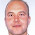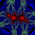# Learning Clojure

## Wednesday, February 16, 2011

### Clojure Dojo 4: Symbolic Differentiation

We've written a fairly good Newton-Raphson solver, but it's a bit hacky to do numerical differentiation when differentiation is so easy by hand.

Here I've tried to create the simplest possible symbolic differentiator.

It relies on four basic rules:

The derivative of a constant is 0.
The derivative of a variable is 1 with respect to itself, 0 with respect to a different variable.
The derivative of a sum is the sum of the derivatives of the parts.
The derivative of a product is the sum of the derivative of the first part times the second part and the first part times the derivative of the second part.

If you tell the computer this then it can differentiate polynomials like
x^5+x^2+2x+3 as long as you express them in terms of binary operators.
`(+ (+ (* x (* x x)) (* 2 (* x x))) 1)`

It's easily extended to deal with arbitrary operators like (* x y z 2), and with functions like sin and cos.

A routine to simplify the answers would also be nice, so that (+ (* 0 (* (* x x) x)) (* x 1)) might be more readably represented as x.

```;; The simplest possible symbolic differentiator

;; Functions to create and unpack additions like (+ 1 2)
(defn make-add [ a b ] (list '+ a b))
(defn addition? [x] (and (=(count x) 3) (= (first x) '+)))
(defn add2   [x] (second (rest x)))

;; Similar for multiplications (* 1 2)
(defn make-mul [ a b ] (list '* a b))
(defn multiplication? [x] (and (=(count x) 3) (= (first x) '*)))
(defn mul1   [x] (second x))
(defn mul2   [x] (second (rest x)))

;; Differentiation.
(defn deriv [exp var]
(cond (number? exp) 0                                                              ;; d/dx c -> 0
(symbol? exp) (if (= exp var) 1 0)                                           ;; d/dx x -> 1, d/dx y -> 0

(multiplication? exp) (make-add (make-mul (deriv (mul1 exp) var) (mul2 exp)) ;; d/dx a*b -> d/dx a * b + a * d/dx b
(make-mul (mul1 exp) (deriv (mul2 exp) var)))
:else :error))

;;an example of use: create the function x -> x^3 + 2x^2 + 1 and its derivative
(def poly '(+ (+ (* x (* x x)) (* 2 (* x x))) 1))

(defn poly->fnform [poly] (list 'fn '[x] poly))

(def polyfn  (eval (poly->fnform poly)))
(def dpolyfn (eval (poly->fnform (deriv poly 'x))))

;;tests

(use 'clojure.test)

(deftest deriv-test
(testing "binary operators"
(is (= (let [m '(* a b)] [(multiplication? m) (make-mul (mul1 m) (mul2 m))]) [true  '(* a b)]))
(testing "derivative function"

(is (= (deriv '0 'x)               '0))
(is (= (deriv '1 'x)               '0))
(is (= (deriv 'x 'x)               '1))
(is (= (deriv 'y 'x)               '0))
(is (= (deriv '(+ x x) 'x)         '(+ 1 1)))
(is (= (deriv '(* x x) 'x)         '(+ (* 1 x) (* x 1))))
(is (= (deriv '(* x x) 'y)         '(+ (* 0 x) (* x 0))))
(is (= (deriv '(* x (* x x)) 'x)   '(+ (* 1 (* x x)) (* x (+ (* 1 x) (* x 1)))))))
(testing "function creation: d/dx (x^3 + 2x^2 + 1) = 3x^2 + 4x "
(let [poly '(+ (+ (* x (* x x)) (* 2 (* x x))) 1)]
(is (= ((eval (poly->fnform poly)) 3) 46))
(is (= ((eval (poly->fnform (deriv poly 'x))) 3))))))

```

1.There's a possible mistake in the addition? clause - I suspect you meant to use add1 and add2 rather than mul1 and mul2. It still works, of course, because addN and mulN do the same things, suggesting you could shorten this by two precious lines using generalised argN functions.

2.Sharp eyes! Well spotted. Will correct.

The little nests of functions for the add/multiply structures are candidates for generic versions.

When we put in subtraction and division this will become more necessary, and if I can find a way of using a macro to do it better than I could with a generic function, that's episode 5....

I was hoping that I could use a macro for defining the function and its derivative from the source code version, but the run-time eval seems to work perfectly well, so it wouldn't be a particularly good example.

3.This comment has been removed by the author.

4.and what about representing polynomies as a vector of its coefficients? (i used it in a prolog homework: https://gist.github.com/795648

5.Javier, that would be very neat and efficient, but the differentiator would only be able to differentiate polynomials then.

This one can differentiate (exp (sin (/ 1 x))) with only small modifications. It actually has a lot in common with an interpreter for a computer language!

I shall add the extra functions for 'the second simplest possible symbolic differentiator' and make a new post. Thanks!

6.I may grab this code some day, once upon a time I started writing something similar... got bored and moved to another project (a Lisp raytracer, I think it was, which sadly is still unfinished too... real work always has preference :/).

Thanks for sharing,

Ruben

7.Nice work! I am looking forward to an expanded version of the symbolic differentiator. Could you explain the `list` and the `'` in `(defn make-add [ a b ] (list '+ a b))` and later on? What is going on there?

8.(ns symdiff.core
(:use [clojure.core.match :only (match)]
clojure.math.numeric-tower))

(defn deriv [exp var]
(match [exp]
[(a :guard number?)] 0
[(a :guard symbol?)] (if (= exp var) 1 0)
[(['+ a b] :seq )] (list '+ (deriv a var) (deriv b var))
[(['* a b] :seq )] (list '+ (list '* (deriv a var) b) (list '* a (deriv b var)))
[(['expt a b] :seq )] (list '* b (list '* (list 'expt a (- b 1)) (deriv a var)))
:else nil))